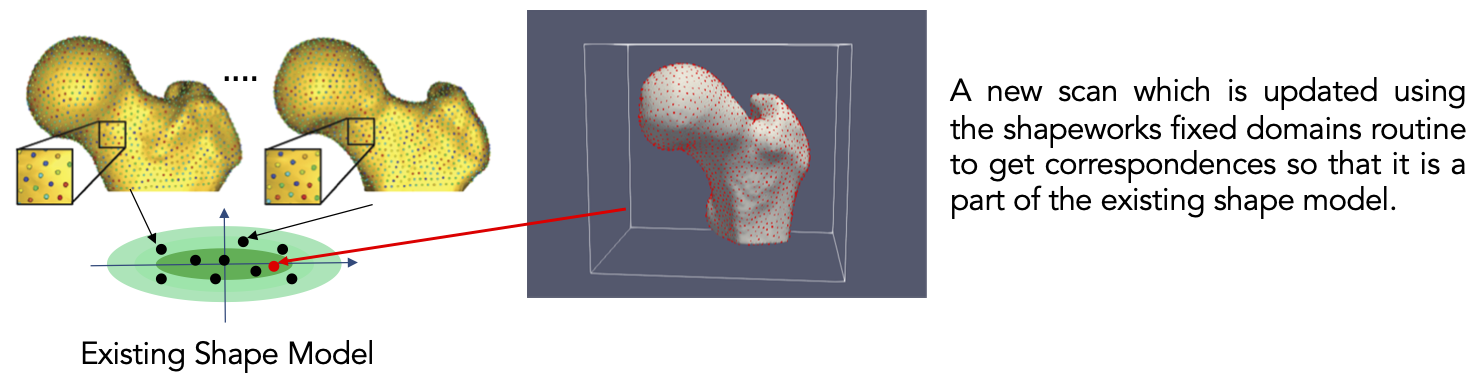# Fixed Domains for Ellipsoid: Correspondences on New Shape

## What and Where is the Use Case?

This use case is designed to demonstrate the functionality of the fixed domain of ShapeWorks. The fixed domains are used for the cases where we need to place correspondences on new shapes using a pre-existing shape model. In this example, we use the example dataset in ellipsoid_fd.zip, which contains a previously generated shape model on ellipsoids and prepped segmentations of two new ellipsoids.The use case is located at: Examples/Python/ellipsoid_fd.py

## Running the Use Case

To run the use case, run RunUseCase.py (in Examples/Python/) with proper tags. The tags control the type of input data and the optimization method. See Getting Started with Use Cases for the full list of tags.

Run the following command to run this use case. This optimization is done only with single-scale support since the new shapes will have the same number of particles as the existing shape model. Hence, there is no need to use the --use_single_scale tag.

$cd /path/to/shapeworks/Examples/Python$ python RunUseCase.py --use_case ellipsoid_fd


This calls ellipsoid_fd.py (in Examples/Python/) to perform the following.

• Loads the existing (pre-trained) shape model and converts the new segmentations into signed distance transforms.
• Optimizes particle distribution (i.e., the shape/correspondence model) on the new shape samples by calling optimization functions in OptimizeUtils.py (in Examples/Python/). See Optimizing Shape Model for details about algorithmic parameters for optimizing the shape model.
• Launches ShapeWorksStudio to visualize the use case results (i.e., optimized shape model on the new shapes and the pre-trained shape model) by calling functions in AnalyzeUtils.py (in Examples/Python/).

If you wish to start with the optimization step using a previously groomed data, add --start_with_prepped_data tag.

\$ python RunUseCase.py --use_case ellipsoid_fd --start_with_prepped_data


## Grooming Data

This use case assumes that the new samples are already aligned with the existing shape model. The only grooming step is computing the signed distance transform for each new segmentation.

## Optimizing Shape Model

Below are the default optimization parameters for this use case. For a description of the optimize tool and its algorithmic parameters, see: How to Optimize Your Shape Model.

        "number_of_particles" : 128,
"use_normals": 0,
"normal_weight": 10.0,
"checkpointing_interval" : 200,
"keep_checkpoints" : 0,
"iterations_per_split" : 100,
"optimization_iterations" : 2000,
"starting_regularization" : 100,
"ending_regularization" : 0.1,
"recompute_regularization_interval" : 2,
"domains_per_shape" : 1,
"domain_type" : 'image',
"relative_weighting" : 10,
"initial_relative_weighting" : 0.01,
"procrustes_interval" : 0,
"procrustes_scaling" : 0,
"save_init_splits" : 0,
"verbosity" : 3,
"number_fixed_domains": len(fileListDT),
"fixed_domain_model_dir": shapemodelDir,
"mean_shape_path": meanShapePath,


In ellipsoid_fd.py (in Examples/Python/), the following is defined.

• fileListDT is the list of distance transforms for the existing shape model
• shapemodelDir is the directory path to the new shape model
• meanShapePath` is the path to the mean (average) shape particles to be used for initializing correspondences on the new shape

## Analyzing Shape Model

ShapeWorksStudio visualizes/analyzes the optimized particle-based shape model by visualizing the mean shape, individual shape samples, and the shape modes of variations. For more information, see: How to Analyze Your Shape Model?.